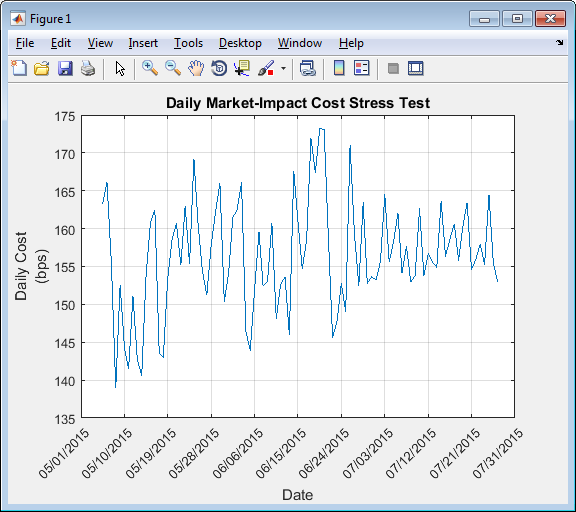Main Content

## Conduct Stress Test on Portfolio

This example shows how to conduct a stress test on a set of stocks using transaction cost analysis from the Kissell Research Group.

• Estimate historical market-impact costs and the corresponding dollar values for the specified date range.

• Use trading costs to screen stocks in a portfolio and estimate the cost to liquidate or purchase a specified number of shares.

• Analyze trading costs during volatile periods of time such as a financial crisis, flash crash, or debt crisis.

To access the example code, enter `edit KRGStressTestingExample.m` at the command line.

### Retrieve Market-Impact Parameters and Load Historical Data

Retrieve the market-impact data from the Kissell Research Group FTP site. Connect to the FTP site using the `ftp` function with a user name and password. Navigate to the `MI_Parameters` folder and retrieve the market-impact data in the `MI_Encrypted_Parameters.csv` file. `miData` contains the encrypted market-impact date, code, and parameters.

```f = ftp('ftp.kissellresearch.com','username','pwd'); mget(f,'MI_Encrypted_Parameters.csv'); close(f) miData = readtable('MI_Encrypted_Parameters.csv','delimiter', ... ',','ReadRowNames',false,'ReadVariableNames',true);```

Load the example data `TradeDataStressTest` from the file `KRGExampleData.mat`, which is included with the Datafeed Toolbox™.

`load KRGExampleData TradeDataStressTest`

For a description of the example data, see Kissell Research Group Data Sets.

Create a Kissell Research Group transaction cost analysis object `k`. Specify initial settings for the date, market-impact code, and number of trading days.

`k = krg(miData,datetime('today'),1,250);`

### Prepare Data for Stress Testing

Specify the date range from May 1, 2015 through July 31, 2015.

```startDate = '5/1/2015'; endDate = '7/31/2015'; ```

Determine the number of stocks `numStocks` in the portfolio. Create a date range `dateRange` from the specified dates. Find the number of days `numDates` in the date range.

```numStocks = length(TradeDataStressTest.Symbol); dateRange = (datenum(startDate):datenum(endDate))'; numDates = length(dateRange); ```

Preallocate the output data table `o`.

```outLength = numStocks*numDates; symbols = TradeDataStressTest.Symbol(:,ones(1,numDates)); sides = TradeDataStressTest.Side(:,ones(1,numDates)); dates = dateRange(:,ones(1,numStocks))'; o = table(symbols(:),sides(:),dates(:),NaN(outLength,1),NaN(outLength,1), ... 'VariableNames',{'Symbol','Side','Date','MI','MIDollar'}); ```

Ensure that the number of shares is a positive value using the `abs` function.

`TradeDataStressTest.Shares = abs(TradeDataStressTest.Shares);`

Convert trade time trade strategy to the percentage of volume trade strategy.

```TradeDataStressTest.TradeTime = TradeDataStressTest.TradeTime ... .* TradeDataStressTest.ADV; TradeDataStressTest.POV = krg.tradetime2pov(TradeDataStressTest.TradeTime, ... TradeDataStressTest.Shares); ```

### Conduct Stress Test by Estimating Historical Market-Impact Costs

Estimate the historical market-impact costs for each stock in the portfolio for the date range using `marketImpact`. Convert market-impact cost from decimal into local dollars. Retrieve the resulting data in the output data table `o`.

```kk = 1; for ii = dateRange(1):dateRange(end) for jj = 1:numStocks k.MiCode = TradeDataStressTest.MICode(jj); k.MiDate = ii; o.MI(kk) = marketImpact(k,TradeDataStressTest(jj,:)); o.MIDollar(kk) = (TradeDataStressTest.Shares(jj) ... * TradeDataStressTest.Price(jj)) ... * o.MI(kk) /10000 * TradeDataStressTest.FXRate(jj); kk = kk + 1; end end```

Display the first three rows of output data.

`o(1:3,:)`
```ans = Symbol Side Date MI MIDollar ______ ____ _________ _____ ________ 'A' 1.00 736085.00 3.84 384.31 'B' 1.00 736085.00 11.43 14292.24 'C' 1.00 736085.00 32.69 20430.65```

The output data contains these variables:

• Stock symbol

• Side

• Historical trade date

• Historical market-impact cost in basis points

• Historical market-impact value in local dollars

Retrieve the daily market-impact cost `dailyCost`. Determine the number of days `numDays` in the output data. Loop through the data and sum the market-impact costs for individual stocks for each day.

```numDays = length(o.Date)/numStocks; idx = 1; for i = 1:numDays dailyCost.Date(i) = o.Date(idx); dailyCost.DailyMiCost(i) = sum(o.MI(idx:idx+(numStocks-1))); idx = idx+numStocks; end```

Display the daily market-impact cost in the specified date range. This figure demonstrates how market-impact costs change over time.

```plot(b.Date,b.DailyMiCost) ylabel({'Daily Cost','(bps)'}) title('Daily Market-Impact Cost Stress Test') xlabel('Date') grid on xData = linspace(b.Date(1),b.Date(92),11); a = gca; a.XAxis.TickLabels = datestr(xData,'mm/dd/yyyy'); a.XTickLabelRotation = 45; ```Kissell, Robert. “Creating Dynamic Pre-Trade Models: Beyond the Black Box.” Journal of Trading. Vol. 6, Number 4, Fall 2011, pp. 8–15.

 Kissell, Robert. “TCA in the Investment Process: An Overview.” Journal of Index Investing. Vol. 2, Number 1, Summer 2011, pp. 60–64.

 Kissell, Robert. The Science of Algorithmic Trading and Portfolio Management. Cambridge, MA: Elsevier/Academic Press, 2013.

 Chung, Grace and Robert Kissell. “An Application of Transaction Costs in the Portfolio Optimization Process.” Journal of Trading. Vol. 11, Number 2, Spring 2016, pp. 11–20.

Download ebook